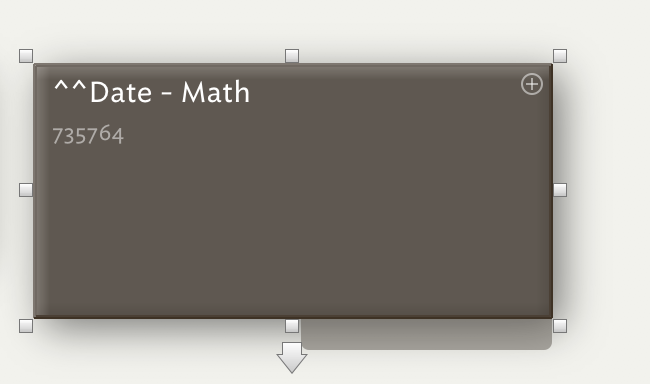# How to do math with Dates

Hello,

I would like to, e.g., estimate the time elapsed between \$EndDate and \$StartDate and display that as the note’s SubTitle. I tried with the following Rule:

``````if(\$EndDate!=0){\$Subtitle=(\$EndDate-\$StartDate)}else{\$Subtitle=today-\$StartDate};
``````

The problem is that the subtraction gives a decimal number rather than the number of years or months that elapsed (see screen capture).I appreciate suggestions on how to address this task.

I apologize if it was discussed elsewhere, but I could not find it.

Thank you very much!

Mariano

I believe this returns the difference in days. The calculation works on the day value, i.e. 25/11/2017 23:50 and 26/11/2017 01:00 are treated in the above context as a difference of 1 (day) although in fact only an hour and 10 minutes apart.

The more formal way of doing this is the days(date1,date2) method. I’d recommend taking a look at my listing of action codes, especially the ones related to Date (Date.etc…) and Interval (Interval.etc…) data types. As with days() there are also years(), months(), hours() and minutes() operators. Note that the Interval type is quite recent and in some cases replaces - or is a more practical alternative - to earlier Date based examples.

Does that help?

If I were doing this, I would use

`if(\$EndDate){\$Subtitle=interval(\$StartDate,\$EndDate)}else{\$Subtitle=interval(\$StartDate,today)};`

Note the first term in the rule. The construct `if(\$EndDate!=0)` returns an indeterminate result because \$EndDate is a date-type attribute, the “zero state” is undefined. What you want to test for is “there is an end date”, that is “exists \$EndDate is True”. Thus `if(\$EndDate)`

The rest of the rule uses the form of the interval operator that compares start and end dates.

Be aware, though, that the interval operator is dynamic – it updates itself continually. So if you used the rule I suggested, the \$Subtitle would be continually updated (watch the seconds in the GIF):This can be interesting**, but it might also be an ongoing computation drain, potentially, if you have a lot of this going on in a file. If that is the case, then the alternate approaches mentioned by @mwra might be more attractive.

** For example, we can watch time tick away as we neglect our chores and never reach the \$EndDate1 Like

Thank you very much @mwra & @PaulWalters. Both approaches worked very well.

I ended up for now with:

``````    if(!\$EndDate)

{if(months(\$StartDate,today)<23)
{\$Subtitle=months(\$StartDate,today)+" months"} else
{\$Subtitle=years(\$StartDate,today)+" years"}}

else

{if(months(\$StartDate,\$EndDate)<23)
{\$Subtitle=months(\$StartDate,\$EndDate)+" months"} else
{\$Subtitle=years(\$StartDate,\$EndDate)+" years"}};

if(!\$StartDate){\$Subtitle="It Never Started!"};
``````

However, I am struggling to understand how to set the precision of the months() function. Adding .precision(0) after months(date1,date2) like so:

``````months(\$StartDate,\$EndDate).precision(0)
``````

returns “0” regardless of the \$StartDate and \$EndDate values.

Thank you very much!

Cheers

Mariano

Actually, I think it is better to define a User Attribute, e.g., \$TimeElapsed (“string”), and then map the \$Subtitle to the attribute like so:

``````if(!\$EndDate)

{if(months(\$StartDate,today)<23)
{\$TimeElapsed=months(\$StartDate,today)+" months"} else
{\$TimeElapsed=years(\$StartDate,today)+" years"}}

else

{if(months(\$StartDate,\$EndDate)<23)
{\$TimeElapsed=months(\$StartDate,\$EndDate)+" months"} else
{\$TimeElapsed=years(\$StartDate,\$EndDate)+" years"}};

if(!\$StartDate){\$TimeElapsed=0; \$Subtitle="It Never Started"} else
{\$Subtitle=\$TimeElapsed};
``````

I still do not know how to define the precision of month().My understanding is that months() returns the number of whole months, i.e. an integer (always rounded down to give complete whole months), and this is what I see testing in the current (v7.3.0) app would confirm that. Therefore you don’t actually don’t need precision.

I can replicate the output you describe, which indicates that although months() returns a number it is in string form. thus is the month difference is 8 then:

`\$SomeNumber = months(\$StartDate,\$EndDate)precision(0);` then \$SomeNumber is `0`

But if we coerce the output to a number:

`\$SomeNumber = months(\$StartDate,\$EndDate).toNumber.precision(0);` then \$SomeNumber is `8`

I need to check with Eastgate but this leads me to suppose all the years(), months(), etc., family of operators output an integer in String a form and not a Number as I’d assumed (but not documented). I’ll check and update documentation as/if needed.

We’re looking into this; it sounds like months() is less assertive than it ought to be that its result is a number.

Thank you very much, @eastgate & @mwra.

Any updates on this? @eastgate, @mwra

Updates on what?

`months()` returning a string rather than a number was fixed in v7.3.1 (see change notes) released c.22 Nov 2017.

1 Like

Thanks for the change notes hint. That helps.

1 Like